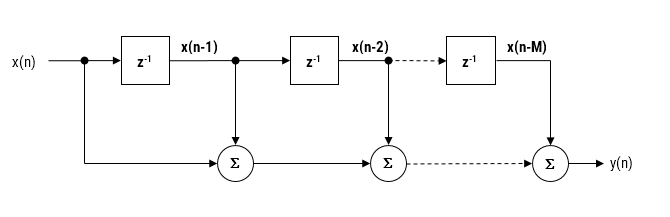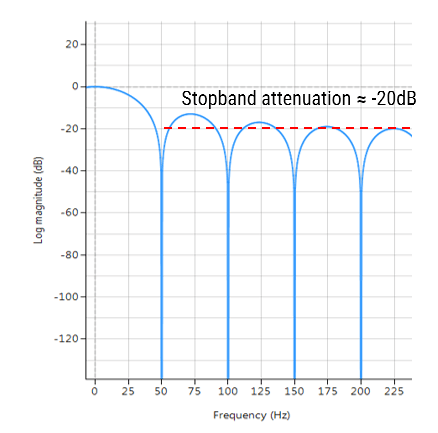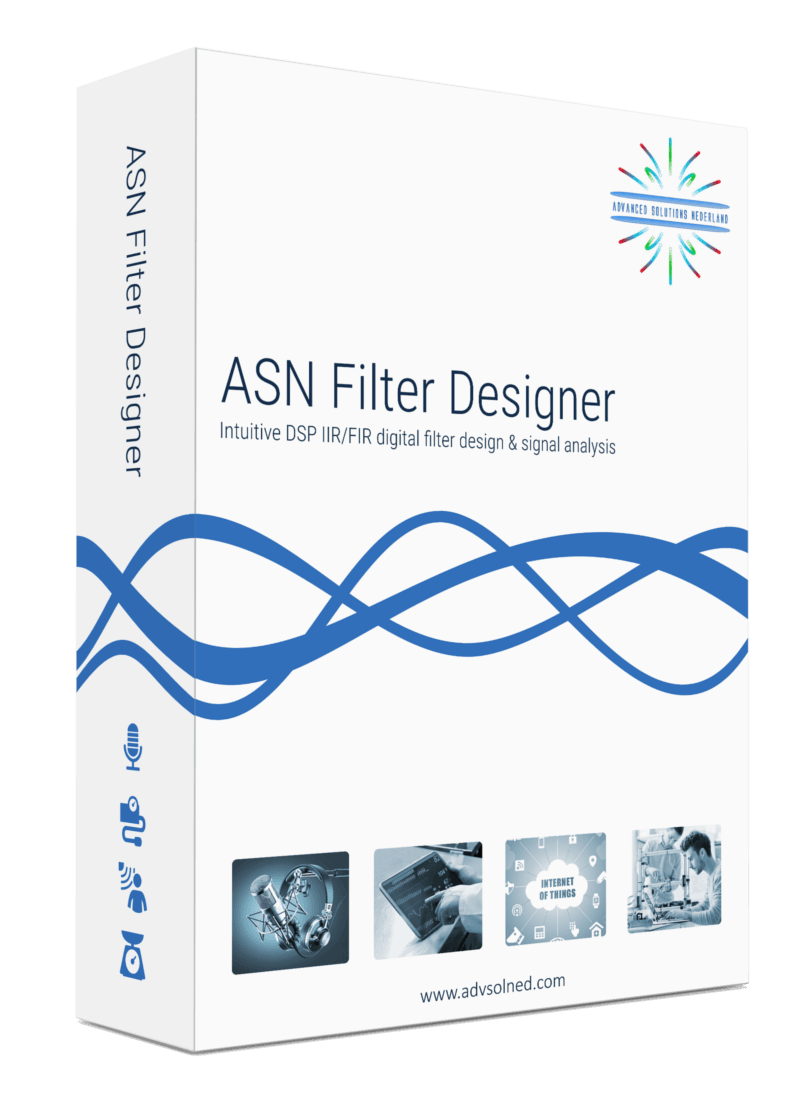## How to export designed IIR/FIR filters to Matlab

For many IoT sensor measurement applications, an IIR or FIR filter is just one of the many components needed for an algorithm. This could be a powerline interference canceller for a biomedical application or even a simpler DC loadcell filter. In many cases, it is necessary to integrate a filter into a complete algorithm in another domain.

Matlab is a well-established numerical computing language developed by the Mathworks that allows for the design of algorithms, matrix data manipulations and data analysis. The product offers a broad range of algorithms and support functions for signal processing applications, and as such is very popular amongst many scientists and engineers worldwide.

## ASN Filter Designer automatic code generator for Matlab

The ASN Filter Designer greatly simplifies exporting a designed filter to Matlab via its automatic code generator. The code generator supports all aspects of the ASN Filter Designer, allowing for a complete design comprised of H1, H2 and H3 filters and math operators to be fully integrated with an algorithm in Matlab.

The Matlab code generator can be accessed via the filter summary options (as shown on the right). Selecting this option will automatically generate a Matlab .m file based on the current design.

A convenient shortcut to the relevant Framework files and examples is available in the Filter summary toolbar via the folder symbol (see left).

Using the three framework files, you may build a demo of your choice based on the exported filter(s) specifications. The framework supports both Real and Complex filters in floating point only.

### Framework files and examples

In order to use the generated code in Matlab without the need for Signal Processing Toolbox, the following three framework files are provided in the ASN Filter Designer’s \Matlab directory:

ASNFDMatlabFilterData.m
ASNFDMatlabImport.m
ASNFDFilter.m

These framework files do not have any special Matlab toolbox dependences, and the example script ASNFDMatlabDemo.m demonstrates the simplicity with which the framework can be integrated into your application for your designed filter. Several example filters generated via the automatic code generator are given within ASNFDMatlabDemo.m in order to get you going!

## Comparing the results to Matlab’s Signal Processing Toolbox

It’s sometimes informative to compare the results of the ASN Filter Designer’s DSP library functions to that of Matlab’s Signal Processing Toolbox.

Designing an IIR Chebyshev Type I filter with the following specifications:

Graphically entering the specifications into the ASN Filter Designer, and fine tuning the design marker positions, the tool automatically designs the filter as a Biquad cascade. Notice that the tool automatically finds the required filter order, and in essence – automatically produces the filter’s exact technical specification!

The frequency response of a 5th order IIR Chebyshev Type I lowpass filter meeting the specifications is shown below:

The resulting filter coefficients are:

Designing the same filter in Matlab using Signal Processing Toolbox:

Fs=500;
Rp=0.1;
Rs=80;
F=2*[25,125]/Fs;

[N,Wn]=cheb1ord(F(1),F(2),Rp,Rs)
[z, p, k] = cheby1(N,Rp,Wn,'low'); % design lowpass

[sos,g]=zp2sos(z,p,k,'up')  % generate SOS form


Running the script, we get the following, where each row of sos is a biquad arranged as:  b0 b1 b2 a0 a1 a2

Analysing both sets of numerator and denominator coefficients, we get exactly the same result! But what about the gain? Matlab outputs a net gain, g = 3.0096e-05 but the ASN Filter Designer optimally assigns a gain to each biquad. Thus, combining the biquad section gains, i.e.  0.078643, 0.013823  and 0.027685 results in a net gain of 3.0096e-05, which is exactly the same net gain as Matlab!

Conclusion: the ASN Filter Designer’s DSP IIR library functions completely match Matlab’s Signal Processing Toolbox results!!

The complete automatically generated code is shown below, where it can be seen that the biquad gains have been pre-multiplied with the feedforward coefficients.

## Using the generated code with Signal Processing Toolbox

If you have Signal Processing Toolbox installed, then you may directly use the generated coefficients given in SOS with the sosfilt() command, e.g.

Clear all;

ASNFD_SOS=[ 0.07864301814, 0.07864301814, 0.00000000000, 1.00000000000,-0.84271396371, 0.00000000000;...
0.01382289248, 0.02764578495, 0.01382289248, 1.00000000000,-1.70536517618, 0.76065674608;...
0.02768538360, 0.05537076720, 0.02768538360, 1.00000000000,-1.79181447713, 0.90255601154;...
];

y=sosfilt(ASNFD_SOS, x); %  x is your input data

plot(x,y); % plot results


As seen, it is as simple as copying and pasting the filter coefficients from the ASN Filter Designer’s filter summary into a Matlab script.

## Drones and DC motor control## Drones and DC motor control – How the ASN Filter Designer can save you a lot of time and effort

Drones are one of the golden nuggets in IoT. No wonder, they can play a pivotal role in congested cities and far away areas for delivery. Further, they can be a great help to give an overview of a large area or places which are difficult or dangerous to reach. However, most of the technology is still in its experimental stage.

Because drones have a lot of sensors, Advanced Solutions Nederland did some research on how drone producing companies have solved questions regarding their sensor technology, especially regarding DC motor control.

### Until now: solutions developed with great difficulty

We found out that most producers spend weeks or even months on finding solutions for their sensor technology challenges. With the ASN Filter Designer, he/she could have come to a solution within days or maybe even hours. Besides, we expect that the measurement would be better too.

The biggest time coster is that until now algorithms were developed by handwork, i.e. they were developed in a lab environment and then tested in real-life. With the result of the test, the algorithm would be tweaked again until the desired results were reached. However, yet another challenge stems from the fact that a lab environment is where testing conditions are stable, so it’s very hard to make models work in real life. These steps result in rounds and rounds of ‘lab development’ and ‘real life testing’ in order to make any progress -which isn’t ideal!

### How the ASN Filter Designer can help save a lot of time and effort

The ASN Filter Designer can help a lot of time in the design and testing of algorithms in the following ways:

• Design, analyse and implement filters for drone sensor applications with real-time feedback and our powerful signal analyser.
• Design filters for speed and positioning control for sensorless BLDC (brushless DC) motor applications.
• Speed up deployment to Arm Cortex-M embedded processors.

### Real-time feedback and powerful signal analyser

One of the key benefits of the ASN Filter Designer and signal analyser is that it gives real-time feedback. Once an algorithm is developed, it can easily be tested on real-life data. To analyse the real-life data, the ASN Filter Designer has a powerful signal analyser in place.

### Design and analyse filters the easy way

You can easily design, analyse and implement filters for a variety of drone sensor applications, including: loadcells, strain gauges, torque, pressure, temperature, vibration, and ultrasonic sensors and assess their dynamic performance in real-time for a variety of input conditions.  With the ASN Filter Designer, you don’t have do to any coding yourself or break your head with specifications: you just have to draw the filter magnitude specification and the tool will calculate the coefficients itself.

### Speed up deployment

Perform detailed time/frequency analysis on captured test datasets and fine-tune your design. Our Arm CMSIS-DSP and C/C++ code generators and software frameworks speed up deployment to a DSP, FPGA or micro-controller.

## An example: designing BLDC motor control algorithms

BLDC (brushless DC) BLDC motors have found use in a variety of application areas, including: robotics, drones and cars. They have significant advantages over brushed DC motors and induction motors, such as: better speed-torque characteristics, high reliability, longer operating life, noiseless operation, and reduction of electromagnetic interference (EMI).

One advantage of BLDC motor control compared to standard DC motors is that the motor’s speed can be controlled very accurately using six-step commutation, making it a good choice for precision motion applications, such as robotics and drones.

### Sensorless back-EMF and digital filtering

For most applications, monitoring of the back-EMF (back-electromotive force) signal of the unexcited phase winding is easier said than done, since it has significant noise distortion from PWM (pulse width modulation) commutation from the other energised windings. The  coupling  between  the  motor parameters, especially inductances, can induce ripple in the back-EMF signal that is synchronous with the PWM commutation.  As a consequence, this induced ripple on the back EMF signal leads to faulty commutation. Thus, the measurement challenge is how to accurately measure the zero-crossings of the back-EMF signal in the presence of PWM signals?

A standard solution is to use digital filtering, i.e. IIR, FIR or even a median (majority) filter. However, the challenge for most designers is how to find the best filter type and optimal filter specification for the motor under consideration.

### The solution

The ASN Filter Designer allows engineers to work on speed and position sensorless BLDC motor control applications based on back-EMF filtering to easily experiment and see the filtering results on captured test datasets in real-time for various IIR, FIR and median (majority filtering) digital filtering schemes. The tool’s signal analyser implements a robust zero-crossings detector, allowing engineers to evaluate and fine-tune a complete sensorless BLDC control algorithm quickly and simply.

So, if you have a measurement problem, ask yourself:

Can I save time and money, and reduce the headache of design and implementation with an investment in new tooling?

Our licensing solutions start from just 125 EUR for a 3-month licence.

Find out what we can do for you, and learn more by visiting the ASN Filter Designer’s product homepage.

## All-pass Peaking/Bell filter

A  Peaking or Bell filter is a type of audio equalisation filter that boosts or attenuates the magnitude of a specified set of frequencies around a centre frequency in order to perform magnitude equalisation. As seen in the plot in the below, the filter gets its name from the shape of the its magnitude spectrum (blue line) which resembles a Bell curve.

Frequency response (magnitude shown in blue, phase shown in purple) of a 2nd order Bell filter peaking at 125Hz.

## All-pass filters

Central to the Bell filter is the so called All-pass filter. All-pass filters provide a simple way of altering/improving the phase response of an IIR without affecting its magnitude response. As such, they are commonly referred to as phase equalisers and have found particular use in digital audio applications.

A second order all-pass filter is defined as:

$$A(z)=\Large\frac{r^2-2rcos \left( \frac{2\pi f_c}{fs}\right) z^{-1}+z^{-2}}{1-2rcos \left( \frac{2\pi f_c}{fs}\right)z^{-1}+r^2 z^{-2}}$$

Notice how the numerator and denominator coefficients are arranged as a mirror image pair of one another.  The mirror image property is what gives the all-pass filter its desirable property, namely allowing the designer to alter the phase response while keeping the magnitude response constant or flat over the complete frequency spectrum.

A Bell filter can be constructed from the $$A(z)$$ filter by the following transfer function:

$$H(z)=\Large\frac{(1+K)+A(z)(1-K)}{2}$$

After some algebraic simplication, we obtain the transfer function for the Peaking or Bell filter as:

$$H(z)=\Large{\frac{1}{2}}\left[\normalsize{(1+K)} + \underbrace{\Large\frac{k_2 + k_1(1+k_2)z^{-1}+z^{-2}}{1+k_1(1+k_2)z^{-1}+k_2 z^{-2}}}_{all-pass filter}\normalsize{(1-K)} \right]$$

• $$K$$ is used to set the gain and sign of the peak
• $$k_1$$ sets the peak centre frequency
• $$k_2$$ sets the bandwidth of the peak

## Implementation

A Bell filter may easily be implemented in ASN FilterScript as follows:

ClearH1;  // clear primary filter from cascade
interface BW = {0,2,0.1,0.5}; // filter bandwidth
interface fc = {0, fs/2,fs/100,fs/4}; // peak/notch centre frequency
interface K = {0,3,0.1,0.5}; // gain/sign

Main()

k1=-cos(2*pi*fc/fs);
k2=(1-tan(BW/2))/(1+tan(BW/2));

Pz = {1,k1*(1+k2),k2}; // define denominator coefficients
Qz = {k2,k1*(1+k2),1}; // define numerator coefficients
Num = (Pz*(1+K) + Qz*(1-K))/2;
Den = Pz;
Gain = 1;


This code may now be used to design a suitable Bell filter, where the exact values of $$K, f_c$$ and $$BW$$ may be easily found by tweaking the interface variables and seeing the results in real-time, as described below.

## Designing the filter on the fly

Central to the interactivity of the FilterScript IDE (integrated development environment) are the so called interface variables. An interface variable is simply stated: a scalar input variable that can be used modify a symbolic expression without having to re-compile the code – allowing designers to design on the fly and quickly reach an optimal solution.

As seen in the code example above, interface variables must be defined in the initialisation section of the code, and may contain constants (such as, fs and pi) and simple mathematical operators, such as multiply * and / divide. Where, adding functions to an interface variable is not supported.

An interface variable is defined as vector expression:

interface name = {minimum, maximum, step_size, default_value};

Where, all entries must be real scalars values. Vectors and complex values will not compile.

All interface variables are modified via the interface variable controller GUI. After compiling the code, use the interface variable controller to tweak the interface variables values and see the effects on the transfer function. If testing on live audio, you may stream a loaded audio file and adjust the interface variables in real-time in order to hear the effects of the new settings.

## FIR Comb Filter: powerline harmonic cancellation and CIC

Comb filters: powerline (50/60Hz) harmonic cancellation filters, audio applications; cascaded integrator–comb (CIC) filters: anti-aliasing, anti-imaging

## The moving average filter

The moving average (MA) filter is perhaps one of the most widely used FIR filters due to its conceptual simplicity and ease of implementation. As seen in the diagram below, notice that the filter doesn’t require any multiplications, just additions and a delay line, making it very suitable for many extreme low-power embedded devices with basic computational capabilities.However, despite its simplicity, the moving average filter is optimal for reducing random noise while retaining a sharp step response, making it a versatile building block for smart sensor signal processing applications.

A moving average filter of length $$L$$ for an input signal $$x(n)$$ may be defined as follows:

$$y(n)=\large{\frac{1}{L}}\normalsize{\sum\limits_{k=0}^{L-1}x(n-k)}$$ for $$\normalsize{n=0,1,2,3….}$$

Where, a simple rule of thumb states that the amount of noise reduction is equal to the square-root of the number of points in the average. For example, an MA of length 9 will result in a factor 3 noise reduction.Frequency response of an MA filter of length 9. Notice the poor stopband attentuation at around -20dB.

• Most commonly used digital lowpass filter.
• Optimal for reducing random noise while retaining a sharp step response.
• Good smoother (time domain).
• Unity valued filter coefficients, no MAC (multiply and accumulate) operations required.
• Conceptually simple to implement.

• Inflexible frequency response: nudging a conjugate zero pair results in non-unity coefficients.
• Poor lowpass filter (frequency domain): slow roll-off and terrible stopband attenuation characteristics.

## Implementation

The MA filter may be implemented in ASN FilterScript as follows:

ClearH1;  // clear primary filter from cascade
Main();
Hd=movaver(8,"symbolic");  // design an 8th order MA
Num = getnum(Hd);   // define numerator coefficients
Den = {1};          // define denominator coefficients
Gain = getgain(Hd); // define gain


A more computationally efficient implementation of the MA filter is discussed here. However, in order to improve the stopband characteristics of the MA, please consider using the Kolmogorov–Zurbenko (KZ) filter.